## Your lab assignment for the week is to measure the amount of charge on the 6.0-cm-diameter metal sphere of a Van de Graaff generator. To do

Question

Your lab assignment for the week is to measure the amount of charge on the 6.0-cm-diameter metal sphere of a Van de Graaff generator. To do so, you’re going to use a spring with spring constant 0.65 N/m to launch a small 1.5 g bead horizontally toward the sphere. You can reliably charge the bead to 2.5 nC, and your plan is to use a video camera to measure the bead’s closest approach to the edge of the sphere as you change the compression of the spring. You’ll then add 3.0-cm, the radius of the sphere, to each to have the distance to the center of the sphere. Your data are as follows:

Compression (cm) Closest approach (cm)
1.6 5.5
1.9 2.6
2.2 1.6
2.5 0.4

Determine the sphere’s charge in nC?

in progress 0
4 months 2021-07-22T00:16:27+00:00 1 Answers 0 views 0

The charge on the sphere is = 309 nC

Explanation:

The electric potential energy is given by the equation: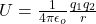where;

Permittivity constant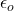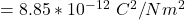the distance between the  two charges = r

The kinetic energy that is said to be given by the spring to the bead is converted to electric potential energy at the closest approach.

So; the kinetic energy is equal to the the elastic potential energy;

The elastic potential energy is represented by the equation: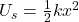where

k = spring constant

x = displacement from the equilibrium position

Converting the charge of the bead from nano coulombs to coulombs ; we have: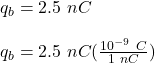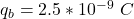Thus, the bead is approaching the metal sphere of Van de Graff generator. Then the kinetic energy is being transferred   to electric potential energy. The process ends when it reaches to the sphere with the Closest approach.

∴ The charge of the sphere can be determined by relating the electric potential energy to elastic potential energy.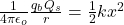Making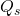the subject pf the formula; we have: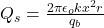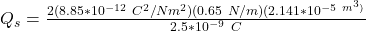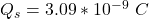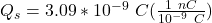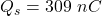Thus the charge on the sphere is = 309 nC